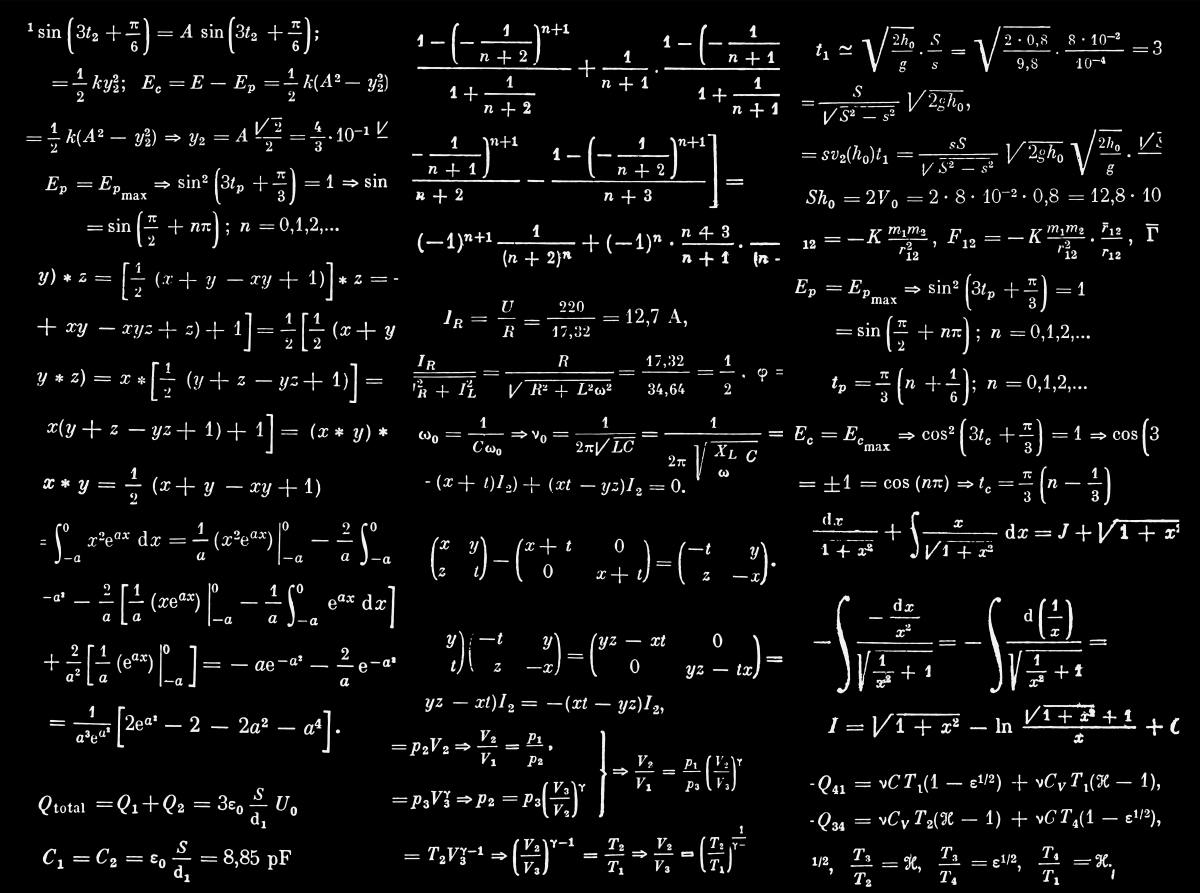# How to Make Calculus Easier: A Fast Way to Find the Derivative of a Function

Sierra is a senior at Marshall University, where she is currently studying Secondary Education Mathematics.Derivatives don't have to be this hard!Dan Cristian Paduret/Unsplash

Here are just a few ways to shorten finding the derivative of a function. You can use these shortcuts for all types of functions, including trig. functions. You will no longer have to use that long definition to find the derivative you need.

I will use D( ) to denote the derivative of ( ).

## Power Rule

The power rule states that D(x^n) = nx^(n-1). You multiply the coefficient by the exponent if there is one. Here are some examples to help you see how it's done.

1. D(x^4) = 4x^3
2. D(5x^8) = 40x^7

You can apply this rule to polynomials as well. Remember: D(f+g) = D(f) + D(g) and D(f-g) = D(f) - D(g)

1. D(6x^3 + 3x^2 + 17) = 18x^2 + 6x
2. D(3x^7 - 5x^3 -23) = 21x^6 - 15x^2
3. D(5x^24 - x^5 + 4x^2) = 120x^23 - 5x^4 + 8x

## Product Rule

The product rule is D(fg) = fD(g) + gD(f). You take the first function and multiply it by the derivative of the second function. You then add that to the first function times the derivative of the first function. Here is an example.

D[(3x^4 + 4x)(12x^2)] = (3x^4 + 4x)D(12x^2) + (12x^2)D(3x^4 + 4x)

D[(3x^4 + 4x)(12x^2)] = (3x^4 + 4x)(24x) + (12x^2)(12x^3 +4)

## Quotient Rule

The quotient rule is D(f/g) = [gD(f) - fD(g)]/ g^2. You take the function on the bottom and multiply it by the derivative of the function on the top. Then you subtract the function of the top multiplied by the derivative of the bottom function. Then you divide all of that by the function on the bottom squared. Here's an example.

Scroll to Continue

## Read More From Owlcation

D[(4x^7)/(8x^3)] = [(8x^3)D(4x^7) - (4x^7)D(8x^3)]/ (8x^3)^2

D[(4x^7)/(8x^3)] = [(8x^3)(28x^6) - (4x^7)(24x^2)]/ (8x^3)^2

## Chain Rule

You use the chain rule when you have functions in the form of g(f(x)). For example, if you were to need to find the derivative of cos(x^2+7), you would need to use the chain rule. An easy way to think about this rule is to take the derivative of the outside and multiply it by the derivative of the inside. Using this example, you would first find the derivative of cosine and then the derivative of what is inside the parenthesis. You would end up with -sin(x^2+7)(2x). I would then clean it up a bit and write it as -2xsin(x^2+7). If you look to the right, you will see a picture of this rule.

Here are some more examples:

D((3x+9x^3)^4) = 4(3x+9x^3)^3 x (3+27x^2) = (12+68x^2)(3x+9x^3)^3

D(sin(4x)) = cos(4x)(4) = 4cos(4x)

## Derivatives to Memorize

Trig Functions

• D(sinx) = cosx
• D(cosx) = -sinx
• D(tanx) = (secx)^2
• D(cscx) = -cscxcotx
• D(secx) = secxtanx
• D(cotx) = -(cscx)^2

Msc.

• D(e^x) = e^x
• D(lnx) = 1/x
• D(constant) = 0
• D(x) = 1

If you have any questions or noticed a mistake in my work please let me know by comment. If you have a specific question on a homework problem that you have don't be afraid to ask; I probably can help. If there is anything else derivative wise that you need help with feel free to ask and I will add it to my post.

Hope this helps!